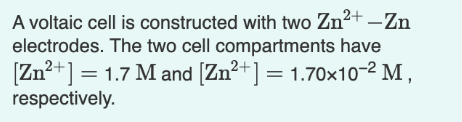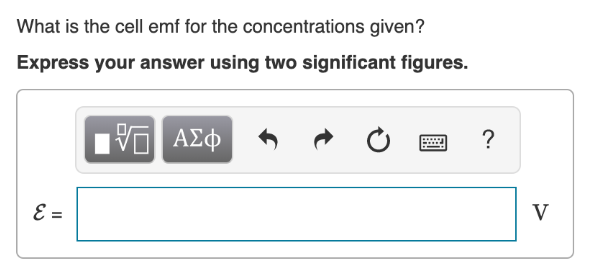# A voltaic cell is constructed with two Zn^2+ -Zn electrodes. The two cell compartments have [Zn^2+] = 1.7 M and [Zn^2+] = 1.70x10^-2M, respectively. What is the cell emf for the concentrations given? Express your answer using two significant figures.